### Home > CALC > Chapter 4 > Lesson 4.2.3 > Problem4-76

4-76.
1.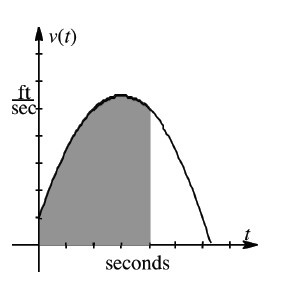The graph at right shows the velocity of an object over time defined by the function v(t) = −0.5t2 + 3t + 1. Homework Help ✎

1. Use your graphing calculator to evaluate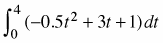.

2. What does the result in part (a) find?

3. What does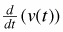represent?

4. What would the units be in part (c)?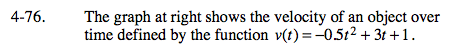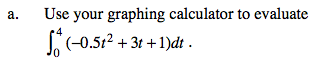$\text{You should get } 17\frac{1}{3}$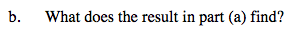You just calculated the area under a velocity graph.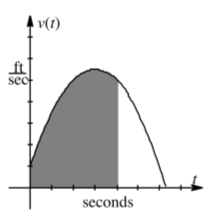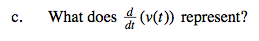Translation 1: What is the derivative of a velocity function?
Translation 2: What is the slope function of a velocity function?

Translation 3: If velocity is the derivative of position, then what is the derivative of velocity?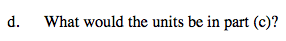$\text{Evaluate and simplify: }\frac{v(t)}{t}, \text{ where }v(t)=\frac{\text{feet}}{\text{seconds}}\text{ and }t=\text{ seconds.}$